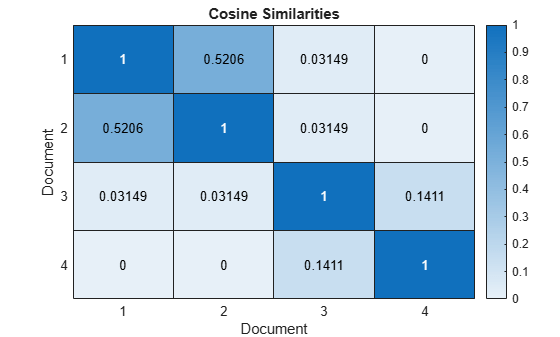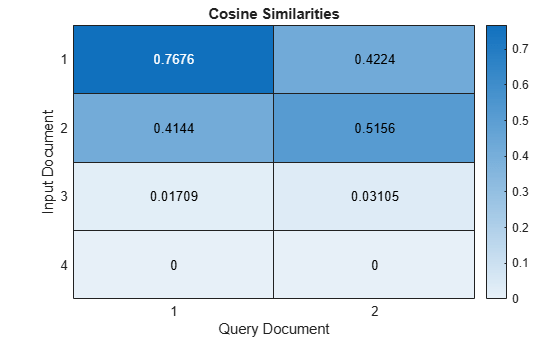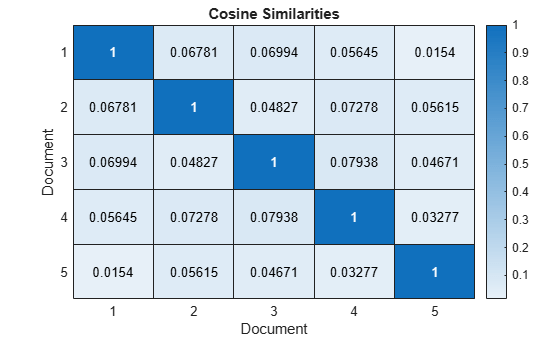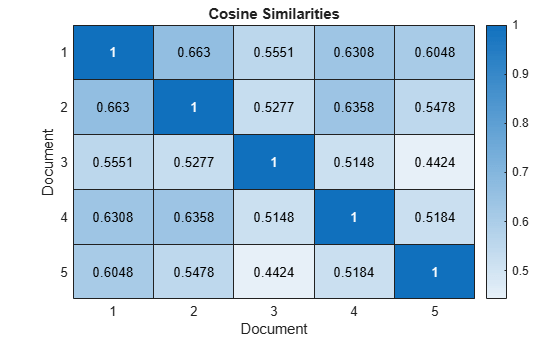# cosineSimilarity

Document similarities with cosine similarity

## Syntax

``similarities = cosineSimilarity(documents)``
``similarities = cosineSimilarity(documents,queries)``
``similarities = cosineSimilarity(bag)``
``similarities = cosineSimilarity(bag,queries)``
``similarities = cosineSimilarity(M)``
``similarities = cosineSimilarity(M1,M2)``

## Description

example

````similarities = cosineSimilarity(documents)` returns the pairwise cosine similarities for the specified documents using the tf-idf matrix derived from their word counts. The score in `similarities(i,j)` represents the similarity between `documents(i)` and `documents(j)`.```

example

````similarities = cosineSimilarity(documents,queries)` returns similarities between `documents` and `queries` using tf-idf matrices derived from the word counts in `documents`. The score in `similarities(i,j)` represents the similarity between `documents(i)` and `queries(j)`.```

example

````similarities = cosineSimilarity(bag)` returns pairwise similarities for the documents encoded by the specified bag-of-words or bag-of-n-grams model using the tf-idf matrix derived from the word counts in `bag`. The score in `similarities(i,j)` represents the similarity between the `i`th and `j`th documents encoded by `bag`.```
````similarities = cosineSimilarity(bag,queries)` returns similarities between the documents encoded by the bag-of-words or bag-of-n-grams model `bag` and `queries` using tf-idf matrices derived from the word counts in `bag`. The score in `similarities(i,j)` represents the similarity between the `i`th document encoded by `bag` and `queries(j)`.```

example

````similarities = cosineSimilarity(M)` returns similarities for the data encoded in the row vectors of the matrix `M`. The score in `similarities(i,j)` represents the similarity between `M(i,:)` and `M(j,:)`.```
````similarities = cosineSimilarity(M1,M2)` returns similarities between the documents encoded in the matrices `M1` and `M2`. The score in `similarities(i,j)` corresponds to the similarity between `M1(i,:)` and `M2(j,:)`.```

## Examples

collapse all

Create an array of tokenized documents.

```textData = [ "the quick brown fox jumped over the lazy dog" "the fast brown fox jumped over the lazy dog" "the lazy dog sat there and did nothing" "the other animals sat there watching"]; documents = tokenizedDocument(textData)```
```documents = 4x1 tokenizedDocument: 9 tokens: the quick brown fox jumped over the lazy dog 9 tokens: the fast brown fox jumped over the lazy dog 8 tokens: the lazy dog sat there and did nothing 6 tokens: the other animals sat there watching ```

Calculate the similarities between them using the `cosineSimilarity` function. The output is a sparse matrix.

`similarities = cosineSimilarity(documents);`

Visualize the similarities between the documents in a heat map.

```figure heatmap(similarities); xlabel("Document") ylabel("Document") title("Cosine Similarities")```Scores close to one indicate strong similarity. Scores close to zero indicate weak similarity.

Create an array of input documents.

```str = [ "the quick brown fox jumped over the lazy dog" "the fast fox jumped over the lazy dog" "the dog sat there and did nothing" "the other animals sat there watching"]; documents = tokenizedDocument(str)```
```documents = 4x1 tokenizedDocument: 9 tokens: the quick brown fox jumped over the lazy dog 8 tokens: the fast fox jumped over the lazy dog 7 tokens: the dog sat there and did nothing 6 tokens: the other animals sat there watching ```

Create an array of query documents.

```str = [ "a brown fox leaped over the lazy dog" "another fox leaped over the dog"]; queries = tokenizedDocument(str)```
```queries = 2x1 tokenizedDocument: 8 tokens: a brown fox leaped over the lazy dog 6 tokens: another fox leaped over the dog ```

Calculate the similarities between input and query documents using the `cosineSimilarity` function. The output is a sparse matrix.

`similarities = cosineSimilarity(documents,queries);`

Visualize the similarities of the documents in a heat map.

```figure heatmap(similarities); xlabel("Query Document") ylabel("Input Document") title("Cosine Similarities")```Scores close to one indicate strong similarity. Scores close to zero indicate weak similarity.

Create a bag-of-words model from the text data in `sonnets.csv`.

```filename = "sonnets.csv"; tbl = readtable(filename,'TextType','string'); textData = tbl.Sonnet; documents = tokenizedDocument(textData); bag = bagOfWords(documents)```
```bag = bagOfWords with properties: Counts: [154x3527 double] Vocabulary: ["From" "fairest" "creatures" "we" ... ] NumWords: 3527 NumDocuments: 154 ```

Calculate similarities between the sonnets using the `cosineSimilarity` function. The output is a sparse matrix.

`similarities = cosineSimilarity(bag);`

Visualize the similarities of the first five documents in a heat map.

```figure heatmap(similarities(1:5,1:5)); xlabel("Document") ylabel("Document") title("Cosine Similarities")```Scores close to one indicate strong similarity. Scores close to zero indicate weak similarity.

For bag-of-words input, the `cosineSimilarity` function calculates the cosine similarity using the tf-idf matrix derived from the model. To compute the cosine similarities on the word count vectors directly, input the word counts to the `cosineSimilarity` function as a matrix.

Create a bag-of-words model from the text data in `sonnets.csv`.

```filename = "sonnets.csv"; tbl = readtable(filename,'TextType','string'); textData = tbl.Sonnet; documents = tokenizedDocument(textData); bag = bagOfWords(documents)```
```bag = bagOfWords with properties: Counts: [154x3527 double] Vocabulary: ["From" "fairest" "creatures" "we" ... ] NumWords: 3527 NumDocuments: 154 ```

Get the matrix of word counts from the model.

`M = bag.Counts;`

Calculate the cosine document similarities of the word count matrix using the `cosineSimilarity` function. The output is a sparse matrix.

`similarities = cosineSimilarity(M);`

Visualize the similarities of the first five documents in a heat map.

```figure heatmap(similarities(1:5,1:5)); xlabel("Document") ylabel("Document") title("Cosine Similarities")```Scores close to one indicate strong similarity. Scores close to zero indicate weak similarity.

## Input Arguments

collapse all

Input documents, specified as a `tokenizedDocument` array, a string array of words, or a cell array of character vectors. If `documents` is not a `tokenizedDocument` array, then it must be a row vector representing a single document, where each element is a word. To specify multiple documents, use a `tokenizedDocument` array.

Input bag-of-words or bag-of-n-grams model, specified as a `bagOfWords` object or a `bagOfNgrams` object. If `bag` is a `bagOfNgrams` object, then the function treats each n-gram as a single word.

Set of query documents, specified as one of the following:

• A `tokenizedDocument` array

• A 1-by-N string array representing a single document, where each element is a word

• A 1-by-N cell array of character vectors representing a single document, where each element is a word

To compute term frequency and inverse document frequency statistics, the function encodes `queries` using a bag-of-words model. The model it uses depends on the syntax you call it with. If your syntax specifies the input argument `documents`, then it uses `bagOfWords(documents)`. If your syntax specifies `bag`, then the function encodes `queries` using `bag` then uses the resulting tf-idf matrix.

Input data, specified as a matrix. For example, `M` can be a matrix of word or n-gram counts or a tf-idf matrix.

Data Types: `double`

## Output Arguments

collapse all

Cosine similarity scores, returned as a sparse matrix:

• Given a single array of tokenized documents, `similarities` is a N-by-N symmetric matrix, where `similarities(i,j)` represents the similarity between `documents(i)` and `documents(j)`, and N is the number of input documents.

• Given an array of tokenized documents and a set of query documents, `similarities` is an N1-by-N2 matrix, where `similarities(i,j)` represents the similarity between `documents(i)` and the `j`th query document, and N1 and N2 represents the number of documents in `documents` and `queries`, respectively.

• Given a single bag-of-words or bag-of-n-grams model, `similarities` is a `bag.NumDocuments`-by-`bag.NumDocuments` symmetric matrix, where `similarities(i,j)` represents the similarity between the `i`th and `j`th documents encoded by `bag`.

• Given a bag-of-words or bag-of-n-grams models and a set of query documents, `similarities` is a `bag.NumDocuments`-by-N2 matrix, where `similarities(i,j)` represents the similarity between the `i`th document encoded by `bag` and the `j`th document in `queries`, and N2 corresponds to the number of documents in `queries`.

• Given a single matrix, `similarities` is a `size(M,1)`-by-`size(M,1)` symmetric matrix, where `similarities(i,j)` represents the similarity between `M(i,:)` and `M(j,:)`.

• Given two matrices, `similarities` is an `size(M1,1)`-by-`size(M2,1)` matrix, where `similarities(i,j)` represents the similarity between `M1(i,:)` and `M2(j,:)`.

## Version History

Introduced in R2020a